Courses

# Methods for Reduction of Delays in Mutlistage Logic Net Electrical Engineering (EE) Notes | EduRev

## Electrical Engineering (EE) : Methods for Reduction of Delays in Mutlistage Logic Net Electrical Engineering (EE) Notes | EduRev

The document Methods for Reduction of Delays in Mutlistage Logic Net Electrical Engineering (EE) Notes | EduRev is a part of Electrical Engineering (EE) category.
All you need of Electrical Engineering (EE) at this link: Electrical Engineering (EE)

Objectives

In this lecture you will learn the following

• Effect of Using Wrong Number of Stages
• Dynamic Latch
• Carry Propagation Gate
• Dynamic Mular C-element
• Fork

24.1 Using Wrong No. of Stages

Let us assume that the number of stages is wrong by a factor s, i.e. the number of stages is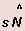. where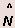is the best number to use. The delay can be expressed as a function of N (assuming parasitic delay of each stage is same as p )as: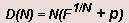Let r be the ratio of the delay when using sN stages to the delay when using best number of stages, N . So,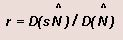Sinceis the best number we know that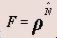. Solving for r we obtain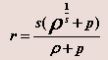This relationship is plotted in the figure for p = 1 and p = 3.59.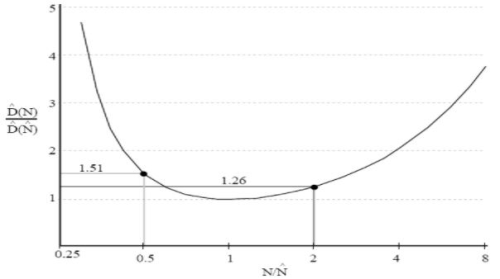Fig 24.11: The relative delay compared to the best possible, as a function of the relative error in the number of stages used

A designer often faces the problem of deciding whether it would be beneficial to change the number of stages in an existing circuit. This can easily be done by calculating the stage effort. If the effort is between 2 and 8, the design is within 35% of best delay. If the effort is between 2.4 and 6, the design is within 15% of best delay. Therefore, there is little benefit in modifying a circuit unless the stage effort is grossly high or low.

24.2 Dynamic Latch

Fig 24.21 shows a dynamic latch: when the clock signal φ is HIGH, and its complement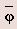is LOW, the gate output q is set to the complement of the input d. The total logical effort of this gate is 4; the logical effort per input for d is 2, and the logical effort of the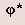bundle is also 2. (Note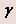is 2)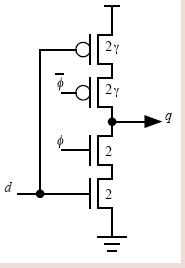Fig 24.21: A dynamic latch with input d and output q. The clock bundle is24.3 Carry Propagation Gate

Fig 24.31 shows one stage of a ripple-carry chain in an adder. The stage accepts carry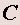in and delivers a carry out in inverted form on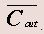. The inputs and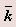come from the two bits to be summed at this stage. The signal is HIGH if this stage generates a new carry, forcing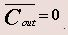. Similarly,is LOW if this stage kills incoming carries, forcing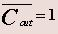The total logical effort of this gate is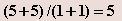. The logical effort per input forin is 2; for the g input it is (1+2)/(1+1); and for theinput it is (2+1)/(1+1).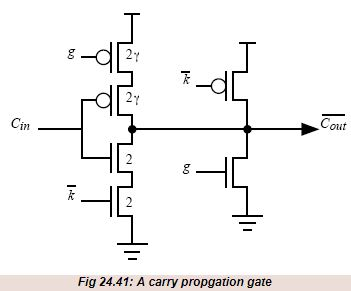24.4 Dynamic Muller C-element
Fig 24.41 shows an inverting dynamic Muller C-element with two inputs. Although this gate is rarely seen in designs for synchronous systems, it is a staple of asynchronous system design. The behavior of the gate is as follows: When both inputs are HIGH, the output goes LOW; when both inputs go LOW, the output goes HIGH. In other conditions, the output retains its previous value - the C-element thus retains state. The total logical effort of this gate is 4, divided between the two inputs.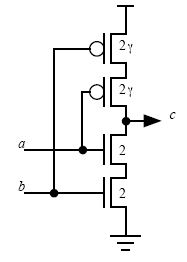Fig 24.41: A two input inverting dynamic Muller C-element

24.5 Fork

If we try to use a signal and an inverter for the complimentary signal then we get unequal delay between two signals. So we use N-stages and adjust the sizing such that we get two complementary signals with equal delay.

Fig 24.51 shows a 2-1 fork and a 3-2 fork, both of which produce the same logic signals.

Fig 24.52 shows a general fork.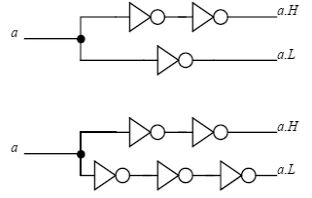Fig 24.51:A 2-1 fork and 3-2 fork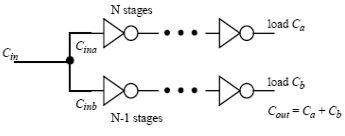Fig 24.52: A general fork

The design of a fork starts out with a known load on the output legs and known total input capacitance. As shown in Fig 24.52, we shall call the two output capacitances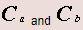. The combined total load driven we will call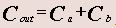. The total input capacitance for the fork we shall call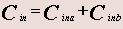, and can thereby describe the electrical effort for the fork as a whole to be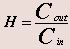. This electrical effort of the fork may differ from the electrical efforts of the individual legs,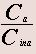and .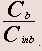The input current to an optimized fork may divide unequally to drive its two legs. Even if the load capacitances on the two legs of the fork are equal, it is not in general true that the input capacitances to the two legs of the fork are equal. Because the legs have different number of amplifiers but must operate with the same delay, their electrical efforts may differ. The leg that can support the larger electrical effort, usually the leg with more amplifiers, will require less input current than the other leg, and can therefore have a smaller input capacitance. If we call the electrical efforts of the two legs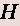a andb, using the notation of Fig 24.52, then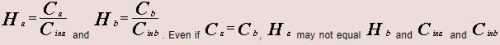may also differ.

The design of a fork is a balancing act. Either leg of the fork can be made faster by reducing its electrical effort, which is done by giving it wider transistors for its amplifier. Doing so, however, takes input current away from the other leg of the fork and will inevitably make it slower. A fixed value of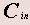provides, in effect, only a certain total width of transistor material to distribute between the first stages of the two legs; putting wider transistors in one leg requires putting narrower transistors in the other leg. The task of designing a minimum delay fork is really the task of allocating the available transistor width set byto the input stages of the two legs.

Offer running on EduRev: Apply code STAYHOME200 to get INR 200 off on our premium plan EduRev Infinity!

,

,

,

,

,

,

,

,

,

,

,

,

,

,

,

,

,

,

,

,

,

;